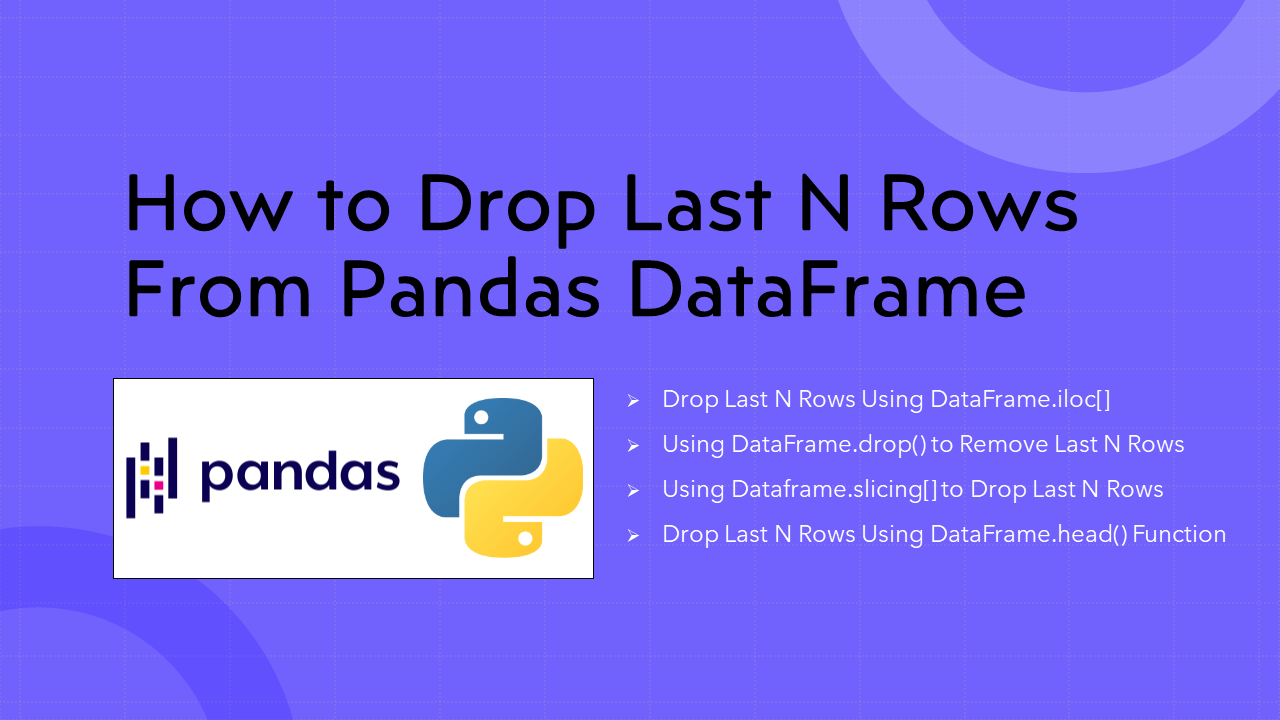# Pandas Drop Last N Rows From DataFrame

• Post author:
• Post category:Pandas

To drop the last `n` rows from the Pandas DataFrame use either `iloc[]`, `drop()`, `slicing[]` and `head()` methods. You can also use the drop() function to drop the rows from the starting point of the DataFrame. In this article, I will explain how to drop/remove the last n rows from Pandas DataFrame with examples.

## 1. Quick Examples of Drop Last N Rows From Pandas DataFrame

If you are in a hurry, below are some quick examples of how to drop the last n rows from DataFrame.

``````
# Below are the quick examples

# Example 1: Number of rows to drop
n = 2

# Example 2: By using DataFrame.iloc[] to drop last n rows
df2 = df.iloc[:-n]

# Example 3: Using drop() function to delete last n rows
df.drop(df.tail(n).index,inplace = True)

# Example 4: Slicing last n rows
df2 = df[:-n]

# Example 5: Using DataFrame.head() function to drop last n rows
``````

Now, let’s create a DataFrame with a few rows and columns, execute these examples, and validate the results. Our DataFrame contains column names `Courses`,` Fee`, `Duration`, and `Discount`.

``````
import pandas as pd
technologies = {
'Courses':["Spark","PySpark","Python","pandas"],
'Fee' :[20000,25000,22000,24000],
'Duration':['30days','40days','35days','60days'],
'Discount':[1000,2300,2500,2000]
}
index_labels=['r1','r2','r3','r4']
df = pd.DataFrame(technologies,index=index_labels)
print("DataFrame:\n", df)
``````

Yields below output.

## 2. Drop Last N Rows Using DataFrame.iloc[]

You can use `DataFrame.iloc[]` the indexing syntax `[:-n]` with n as an integer to select the rows excluding the last n rows from the pandas DataFrame which results in a drop of the last n rows. You can also use iloc[] to drop rows by Index from pandas DataFrame.

``````
# By using DataFrame.iloc[] to drop last n rows
n = 2
df2 = df.iloc[:-n]
print("After dropping last n rows:\n", df2)
``````

Yields below output.

## 3. Use DataFrame.drop() to Remove Last N Rows

By using `DataFrame.drop()` method you can remove the last n rows from pandas DataFrame. Use `index` param to specify the last index and `inplace=True` to apply the change on the existing DataFrame. For instance, `df.drop(df.tail(n).index,inplace=True)`.

``````
# Using drop() function to delete last n rows
n = 3
df.drop(df.tail(n).index,inplace = True)
print(df)
``````

Yields below output.

``````
# Output:
Courses    Fee Duration  Discount
r1   Spark  20000   30days      1000
``````

## 4. Using Dataframe.slicing[] to Drop Last N Rows

Alternatively, You can also use `df[:-n]` to slice the last n rows of pandas DataFrame.

``````
# Slicing last n rows
n = 2
df2 = df[:-n]
print(df2)
``````

Yields below output.

``````
# Output:
Courses    Fee Duration  Discount
r1    Spark  20000   30days      1000
r2  PySpark  25000   40days      2300
``````

## 5. Drop Last N Rows Using DataFrame.head() Function

You can also use `df.head(-n)` to delete the last n rows of pandas DataFrame. Generally, `DataFrame.head()` function is used to show the first n rows of a pandas DataFrame but you can pass a negative value to skip the rows from the bottom.

``````
# Using DataFrame.head() function to drop last n rows
n = 2
print(df2)
``````

Yields the same output as above.

## 6. Complete Example For Drop Last N Rows From DataFrame

``````
import pandas as pd
technologies = {
'Courses':["Spark","PySpark","Python","pandas"],
'Fee' :[20000,25000,22000,24000],
'Duration':['30days','40days','35days','60days'],
'Discount':[1000,2300,2500,2000]
}
index_labels=['r1','r2','r3','r4']
df = pd.DataFrame(technologies,index=index_labels)
print(df)

# Number of rows to drop
n = 2
# By using DataFrame.iloc[] to drop last n rows
df2 = df.iloc[:-n]
print(df2)

#  Number of rows to drop
n = 1
# Using drop() function to delete last n rows
df.drop(df.tail(n).index,
inplace = True)
print(df)

# Number of rows to drop
n = 2
# Slicing last n rows
df2 = df[:-n]
print(df2)

#  Number of rows to drop
n = 2
#  Using DataFrame.head() function to drop last n rows
print(df2)
``````

## Conclusion

In this article, you have learned how to drop the last n rows From Pandas DataFrame using DataFrame.iloc[], `DataFrame.drop()`, `DataFrame.head() `and `Dataframe.slicing[]` function with examples.

Happy Learning !!

## References

### Naveen

I am a Data Engineer with 20+ years of experience in transforming data into actionable insights. Over the years, I have honed my expertise in designing, implementing, and maintaining data pipelines with frameworks like Apache Spark, PySpark, Pandas, R, Hive and Machine Learning. My journey in the field of data engineering has been a continuous learning, innovation, and a strong commitment to data integrity. I have started this SparkByExamples.com to share my experiences with the data as I come across. You can learn more about me at LinkedIn